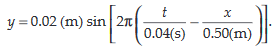# The equation of a wave on a string of linear mass density of 0.04 kg m-1 is given by What is the tension in the string?

The equation of a wave on a string of linear mass density of 0.04 kg m-1 is given byWhat is the tension in the string?

Anonymous User Physics Waves and Sound Waves 12 May, 2020 62 views